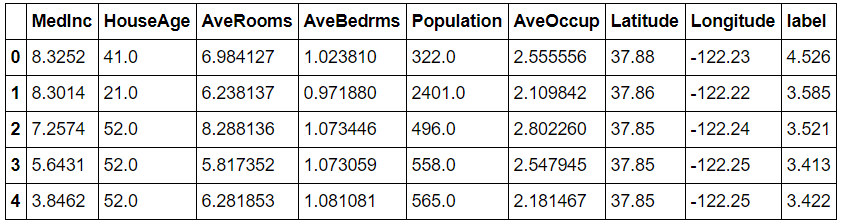# Introduction

Tensorflow is a numerical computation library that provides many features for defining your computation. It represents the mathematical operations as nodes in a graph essentially forming a data flow graph. But these are quite low level and requires quite a lot of experience and code. It also provides several high level APIs that allow us to quickly build a computational model on top of it. Estimator is one of them that simplifies machine learning programming. It allows us to quickly build a model, train, evaluate and export for use in production.Estimator API provides pre-made models like linear regression model, deep neural network based classifier, decision trees etc. In this tutorial we’ll train and evaluate LinearRegressor to predict house prices.

# Data

For the dataset, we’ll be using California housing dataset. We’ll also split the dataset into training and evaluation set.

``````1
2
3
4
5
6
7
8
9
10
11
12
from sklearn.datasets.california_housing import fetch_california_housing
import pandas as pd
import numpy as np

dataset = fetch_california_housing()
df = pd.DataFrame(dataset.data, columns=dataset.feature_names)
df['label'] = dataset.target

p = np.random.normal(size=(len(df))) < 0.7
train_df = df[p]
eval_df = df[~p]
print(len(train_df), len(eval_df))
``````Total training set include 15639 samples and evaluation set contains 5001 samples.

# Using Estimator API

We’ll need to do the following to use pre-made Estimators:

1. create input function
2. define feature columns
3. initialize a model
4. train the model

## Creating input functions

Input functions are used to return data for training, evaluating and prediction. There are two utility functions provided by tensorflow in tf.estimator.inputs module to create input functions. numpy_input_fn returns an input function that feeds a dictionary of numpy arrays while pandas_input_fn returns an input function that feeds a Pandas dataframe to the model. We’ll use pandas_input_fn to create our input function.

``````1
2
3
4
5
6
7
8
def get_input_function(df, num_epochs):
return tf.estimator.inputs.pandas_input_fn(x=df,
y=df['label'],
batch_size=128,
num_epochs=num_epochs,
shuffle=True,
queue_capacity=1000, # size of read queue
)
``````

## Feature columns

Next, we need to define the feature columns we want to use in our model. A feature column is an object describing how the model should use the raw input data. There are many options in _tf.feature_column_module to represent the data. For this demo, we’ll only use median income to predict the price, so we define a numeric column named ‘MedInc’ as one of our features. If we intent to use other, we can add them to the list.

``````1
2
3
4
5
def get_feature_columns():
return [
tf.feature_column.numeric_column('MedInc'),
# many others if we use it
]
``````

Note that even though we passed the whole dataframe to x parameter in our get_input_function, the model will only use the columns that we specify in the feature columns.

## Create/train/evaluate estimator

Not we can create our estimator and train it. We’ll create a Linear model and define training and evaluation specs. Then we can call train_and_evaluate function. It takes care of training, evaluating, creating model checkpoints all for free! We can also individually train and evaluate by calling estimator.train and estimator.evaluate functions respectively.

``````1
2
3
4
5
6
7
8
9
10
11
12
def train_and_evaluate(output_dir, num_train_steps):
estimator = tf.estimator.LinearRegressor(feature_columns=get_feature_columns(), model_dir=output_dir)
train_spec = tf.estimator.TrainSpec(input_fn=get_input_function(train_df, num_epochs=8),
max_steps=num_train_steps,
)
eval_spec = tf.estimator.EvalSpec(input_fn=get_input_function(eval_df, num_epochs=1), # use eval dataset
steps=None, # evaluate until input function raises end-of-input exception
start_delay_secs=1, # start evaluating after waiting for 1 seconds
throttle_secs=10, # evaluate every 10 seconds
)
tf.estimator.train_and_evaluate(estimator, train_spec, eval_spec)
train_and_evaluate("./output", 5000)
``````

In the previous step, we specified model_dir parameter to create checkpoints. When we want to load the model we can use the checkpoint data to load the state of our model at that instant. We can pass model_dir parameter when creating the estimator and all the trained parameters of the model will be loaded so that we can make predictions or resume training.
To predict we can use predict function of the estimator which returns a Python generator. The generator can either return the predictions one by one or in bulk. This behaviour is defined by yield_single_examples parameter. If you set yield_single_examples to True then, it will yield predictions for batch_size number of rows that we’ve defined in get_input_function. In this case, it will yield 128 predictions at a time since our batch_size is 128. Another thing to note is that the predict function returns a dictionary and the predicted values in a numpy column vector i.e. a matrix with dimensions batch_size x 1 so we use flatten function to convert it to a normal array.

``````1
2
3
4
5
6
7
8
estimator = tf.estimator.LinearRegressor(feature_columns=get_feature_columns(), model_dir="./output")
estimator.evaluate(get_input_function(eval_df, 1))
# {'average_loss': 0.7667341, 'global_step': 5000, 'loss': 95.86093}

predictions = []
for p_dict in estimator.predict(get_input_function(eval_df, 1), yield_single_examples=False):
# p_dict is a dictionary with a 'predictions' key
predictions.extend(p_dict['predictions'].flatten())
``````

# Conclusion

To summarize, Estimator API provides a high level api for building machine learning models that reduces a lot of boilerplate code. In future posts, we’ll use the Estimator APIs to train a deep neural network locally as well as in the cloud. We’ll also deploy our trained model in Google cloud so that it can be accessed via REST API.

Categories:

Updated: